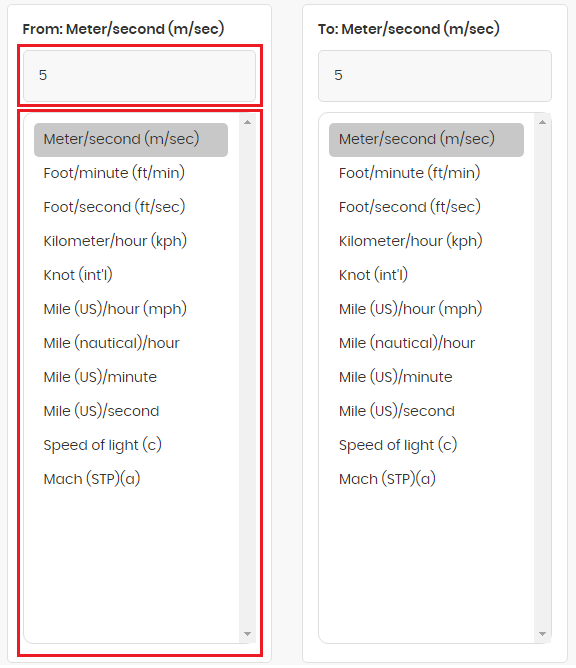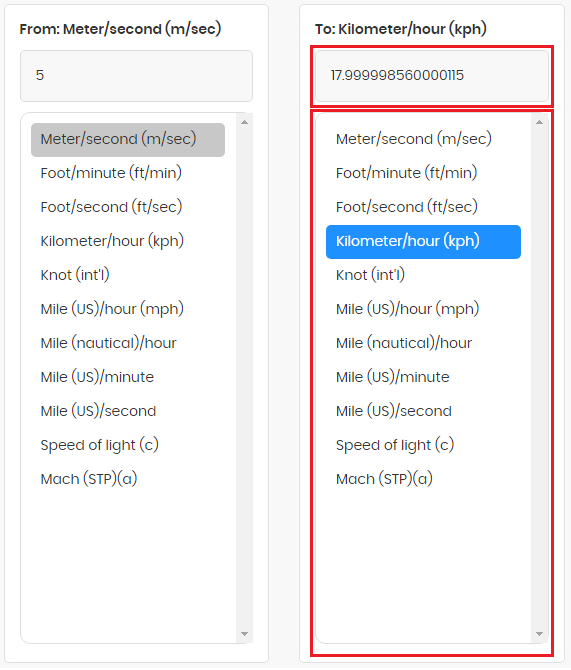# Speed Converter

Solve your problems related to speed and make evaluations based on your calculations with the assistance of speed converter. To compute, add value in the text field found above the first pole of units and the conversion value will be provided as soon as you tap on a unit located in the column of units.

From: Meter(m)
To: Meter(m)

Speed and velocity, where one is measured with a direction, and the other one is calculated on the basis of the position of the object are both vital when making computations. According to the system of units meter per second is the derived unit for both. To measure the speed means how fast a thing is moving you, and you will need a simple formula which is distance divided by time. Linear calculations are required when an item is in motion on a straight line whereas velocity asks for a direction.

You can put all your theoretical measurements in our Speed Calculator to compute time, speed, and distance with accuracy. All the speed and velocity of vehicles like cars, bicycles, etc. from one position to another can be calculated by the bike Speed Converter.

Other commonly used units are kilometers and miles per hour in case you need to find speed or velocity of things that cover a long distance. If we measure things with direction, then it will become velocity. We are all aware of the commonly used terms like the speed of light, sound, etc. and have used them in calculations in our studies. If there were no accuracy in calculating speed and velocity, people would never have made it to space. They would never know, how fast an object needs to be to cover the distance between cities.

The vector is used when a direction is added to the course and scalar is the distance on a straight path. You can measure how much speed will be required by your foot or vehicle to cover the distance with our time speed and distance calculator. Using the program is actually pretty easy if you follow the below-given instructions you will have your answer in a matter of seconds.

1. On the main page of wind speed calculator, you will find a couple of units in two windows. In the first window and text field below the “From” title, you will write the number and select its current unit, which needs to be transformed, as shown in the picture below.
2.3. In the second window below the title “To” as soon as you click on the unit in which you wish to turn your value, the results will be displayed immediately with no further conditions to wait.
4.The most commonly used type of speeds in our subjects is light, sound, four-velocity, and hypervelocity. We are aware of the speed of light in a vacuum is the fastest, and it is nearly impossible to match that speed because it will ask for power that is impossible to generate.

However, the speed of sound can be easily calculated, and today, the airplanes and other flying objects can have a speed close to the speed of sound but still unmatchable. The planes today that is installed with turbofan technology, their speed ranges between 240 to 260 meters per second, but again, it is almost hundred meters per second less than the speed of sound in air which is around 340 m/s

Whereas four-velocity is required for computations in space-time.

The object with this velocity moves with speed of light, which is sustained but has a difference of direction. Then there is the hypervelocity which comes after four-velocity and is calculated for things that are faster or equal to three thousand meters per second. This type of speed is required to be measured for astronauts because tiny asteroids in space can have a significant effect over spaceships plus items when reaching the maximum hyper velocity, they turn to gas when a collision takes place.

Facilities that build space suits and air crafts have tech advanced enough to test and prepare their objects for such occurrences. At these places, they speed up the little items up to eight thousand meters per second to check how the things are going to strike and what kind of effect it will bring on their shields.